International
Tables for
Crystallography
Volume B
Reciprocal space
Edited by U. Shmueli

International Tables for Crystallography (2006). Vol. B, ch. 2.4, pp. 264-265   | 1 | 2 |

## Section 2.4.2. Isomorphous replacement method

M. Vijayana* and S. Ramaseshanb

aMolecular Biophysics Unit, Indian Institute of Science, Bangalore 560 012, India, and bRaman Research Institute, Bangalore 560 080, India
Correspondence e-mail:  mv@mbu.iisc.ernet.in

### 2.4.2. Isomorphous replacement method

| top | pdf |

#### 2.4.2.1. Isomorphous replacement and isomorphous addition

| top | pdf |

Two crystals are said to be isomorphous if (a) both have the same space group and unit-cell dimensions and (b) the types and the positions of atoms in both are the same except for a replacement of one or more atoms in one structure with different types of atoms in the other (isomorphous replacement) or the presence of one or more additional atoms in one of them (isomorphous addition). Consider two crystal structures with identical space groups and unit-cell dimensions, one containing N atoms and the other M atoms. The N atoms in the first structure contain subsets P and Q whereas the M atoms in the second structure contain subsets P, Q′ and R. The subset P is common to both structures in terms of atomic positions and atom types. The atomic positions are identical in subsets Q and Q′, but at any given atomic position the atom type is different in Q and Q′. The subset R exists only in the second structure. Ifanddenote the structure factors of the two structures for a given reflection,and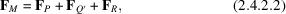where the quantities on the right-hand side represent contributions from different subsets. From (2.4.2.1)and (2.4.2.2)we have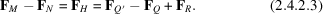The above equations are illustrated in the Argand diagram shown in Fig. 2.4.2.1.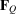andwould be collinear if all the atoms in Q were of the same type and those in Q′ of another single type, as in the replacement of chlorine atoms in a structure by bromine atoms.Figure 2.4.2.1 | top | pdf |Vector relationship betweenand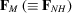.

We have a case of isomorphous replacement' if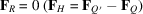and a case of isomorphous addition' if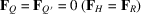. Onceis known, in addition to the magnitudes ofand, which can be obtained experimentally, the two cases can be treated in an equivalent manner in reciprocal space. In deference to common practice, the term isomorphous replacement' will be used to cover both cases. Also, in as much asis the vector sum ofand,andwill be used synonymously. Thus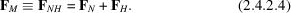#### 2.4.2.2. Single isomorphous replacement method

| top | pdf |

The number of replaceable (or added') atoms is usually small and they generally have high atomic numbers. Their positions are often determined by a Patterson synthesis of one type or another (see Chapter 2.3). It will therefore be assumed in the following discussion thatis known. Then it can be readily seen by referring to Fig. 2.4.2.2that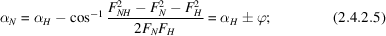whenis derived from its cosine function, it could obviously be positive or negative. Hence, there are two possible solutions for. These two solutions are distributed symmetrically about. One of these would correspond to the correct value of. Therefore, in general, the phase angle cannot be unambiguously determined using a pair of isomorphous crystals.Figure 2.4.2.2 | top | pdf |Relationship between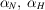and.

The twofold ambiguity in phase angle vanishes when the structures are centrosymmetric.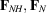andare all real in centric data and the corresponding phase angles are 0 or. From (2.4.2.4)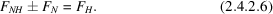The sign ofis already known and the signs ofand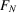can be readily determined from (2.4.2.6)(Robertson & Woodward, 1937).

When the data are acentric, the best one can do is to use both the possible phase angles simultaneously in a Fourier synthesis (Bokhoven et al., 1951). This double-phased synthesis, which is equivalent to the isomorphous synthesis of Ramachandran & Raman (1959), contains the structure and its inverse when the replaceable atoms have a centrosymmetric distribution (Ramachandran & Srinivasan, 1970). When the distribution is noncentrosymmetric, however, the synthesis contains peaks corresponding to the structure and general background. Fourier syntheses computed using the single isomorphous replacement method of Blow & Rossmann (1961) and Kartha (1961) have the same properties. In this method, the phase angle is taken to be the average of the two possible solutions of, which is alwaysor. Also, the Fourier coefficients are multiplied by cos, following arguments based on the Blow & Crick (1959) formulation of phase evaluation (see Section 2.4.4.4). Although Blow & Rossmann (1961) have shown that this method could yield interpretable protein Fourier maps, it is rarely used as such in protein crystallography as the Fourier maps computed using it usually have unacceptable background levels (Blundell & Johnson, 1976).

#### 2.4.2.3. Multiple isomorphous replacement method

| top | pdf |

The ambiguity inin a noncentrosymmetric crystal can be resolved only if at least two crystals isomorphous to it are available (Bokhoven et al., 1951). We then have two equations of the type (2.4.2.5), namely,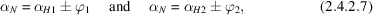where subscripts 1 and 2 refer to isomorphous crystals 1 and 2, respectively. This is demonstrated graphically in Fig. 2.4.2.3with the aid of the Harker (1956) construction. A circle is drawn withas radius and the origin of the vector diagram as the centre. Two more circles are drawn with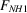and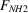as radii and the ends of vectors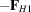and, respectively as centres. Each of these circles intersects thecircle at two points corresponding to the two possible solutions. One of the points of intersection is common and this point defines the correct value of. With the assumption of perfect isomorphism and if errors are neglected, the phase circles corresponding to all the crystals would intersect at a common point if a number of isomorphous crystals were used for phase determination.Figure 2.4.2.3 | top | pdf |Harker construction when two heavy-atom derivatives are available.

### References

Blow, D. M. & Crick, F. H. C. (1959). The treatment of errors in the isomorphous replacement method. Acta Cryst. 12, 794–802.
Blow, D. M. & Rossmann, M. G. (1961). The single isomorphous replacement method. Acta Cryst. 14, 1195–1202.
Blundell, T. L. & Johnson, L. N. (1976). Protein crystallography. London: Academic Press.
Bokhoven, C., Schoone, J. C. & Bijvoet, J. M. (1951). The Fourier synthesis of the crystal structure of strychnine sulphate pentahydrate. Acta Cryst. 4, 275–280.
Harker, D. (1956). The determination of the phases of the structure factors of non-centrosymmetric crystals by the method of double isomorphous replacement. Acta Cryst. 9, 1–9.
Kartha, G. (1961). Isomorphous replacement in non-centrosymmetric structures. Acta Cryst. 14, 680–686.
Ramachandran, G. N. & Raman, S. (1959). Syntheses for the deconvolution of the Patterson function. Part I. General principles. Acta Cryst. 12, 957–964.
Ramachandran, G. N. & Srinivasan, R. (1970). Fourier methods in crystallography. New York: Wiley–Interscience.
Robertson, J. M. & Woodward, I. (1937). An X-ray study of the phthalocyanines. Part III. Quantitative structure determination of nickel phthalocyanine. J. Chem. Soc. pp. 219–230.